﻿ QSAR Studies of the Antifungal Activities of α-Diaminophosphonates Derived from Dapsone by DFT MethodPublications are Open
Access in this journal
Article Versions
Export Article
• Normal Style
• MLA Style
• APA Style
• Chicago Style
Research Article
Open Access Peer-reviewed

### QSAR Studies of the Antifungal Activities of α-Diaminophosphonates Derived from Dapsone by DFT Method

Jean Stéphane N’dri, Ahmont Landry Claude Kablan, Bafétigué Ouattara, Mamadou Guy-Richard Koné , Lamoussa Ouattara, Charles Guillaume Kodjo, Nahossé Ziao
Journal of Materials Physics and Chemistry. 2019, 7(1), 1-7. DOI: 10.12691/jmpc-7-1-1
Received December 02, 2018; Revised January 09, 2019; Accepted January 22, 2019

### Abstract

This QSAR study focused on a series of α-diaminophosphonates derived from Dapsone. Three models have been obtained by taking into account both molecular descriptors and antifungal activities such as those of Aspergillus niger, Aspergillus foetidus and Fusarium oxysporum. Quantum chemistry methods were used at B3LYP/6-31G (d) calculation level to obtain the molecular descriptors. The statistical indicators of the first model which talk about Aspergillus niger activity are: the determination coefficient R2 = 0.976, the standard deviation S = 0.034, the Fischer test F = 80.857 and the correlation coefficient of the cross-validation Q2CV = 0.975. Those of the second model which highlight Aspergillus foetidus activity are: regression coefficient R2 = 0.946, standard deviation S = 0.041, Fischer test F = 35.353 and cross-validation correlation coefficient Q2CV = 0.943. The statistical indicators of the third model are: the determination coefficient R2 = 0.931, the standard deviation S = 0.065, the Fischer F = 27.043 and the correlation coefficient of the cross-validation Q2CV = 0.926. This last model talks about Fusarium oxysporum activity. These models, according to the values of their statistical descriptors, possess good statistical performance. The quantum descriptors such as global electronegativity (χ), the energy of the highest occupied molecular orbital (EHO) and electronic energy (ε0) are responsible for the biological activities of the studied Dapsone derivatives. In addition, electronic energy and global electronegativity are proved to be the priority descriptors in predicting the antifungal activities of these Dapsone derivatives. The acceptance criteria of Eriksson et al. used for the test set are verified. The values of the ratio of theoretical and experimental activities for the validation set tend towards unity.

### 1. Introduction

The fight against infectious diseases remains a paramount public health issue; this can be explained by both high mortality and morbidity rate which are associated to these diseases 1, 2. In addition, anti-infective chemotherapies are currently experiencing a lack of efficacy due mainly to the drug resistance phenomena of infectious germs 3. In such a context, the continued development of new high-performance (more powerful) drugs is a vital necessity. This QSAR study focuses on a series of ten (10) molecules derived from Dapsone. Diamino-diphenyl sulfone or DDS (Dapsone) is a biologically active sulfone (bacteriostatic), used in the treatment of leprosy, which presents to date many undesirable effects (skin, neurological and psychiatric) and resistance during treatment 4. It has therefore been envisaged by some chemists the synthesis of Dapsone derivatives in order to produce compounds with more interesting biological activities 5, 6, 7. The development of quantitative structure-activity relationship studies (QSAR), knows a particular boom and has become essential in pharmaceutical chemistry and for drug design 8. This study is used to limit the excessive number of experiments which are sometimes long and expensive and it reduces the cost of drug production for pharmaceutical companies 9, 10. α-aminophosphonates are important biologically active compounds and structural analogs of natural amino acids 11. They have received considerable attention in medicinal, organic and chemical products. Many of these derivatives have applications as antibiotics 12, herbicides 13, fungicides, plant growth regulators 14, antithrombotic agents 15, peptidases, proteinases 16, and enzyme inhibitors 17. They can also suppress the growth of various tumors and viruses 18.

The main objective of this work is to apply Quantitative Structure-Activity Relationships (QSAR) modeling to develop reliable models for the prediction of antifungal activities in a series of ten (10) α-diaminophosphonates derived from Dapsone (Figure 1).

• Figure 1. Molecular structures of α-diaminophosphonates used for QSAR models

### 2. Materials and Methods

2.1. Data Source

The Dapsone derivatives that are the subject of this study were synthesized and tested by Subba et al 19 for their antifungal activities. The ten (10) molecules used in this study have Minimum Inhibitory Concentrations (MICs) ranging from 12.0 to 50.0 μg/mL. The Minimum Inhibitory Concentration (MIC) represents the lowest concentration that has given an antifungal response. Biological data are generally expressed as the opposite of the decimal logarithm of activity (-log10(C)) to obtain better mathematical values when structures are biologically active 20, 21. The antifungal activity will be expressed by the antifungal potential pMIC defined by equation (1):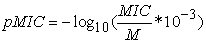(1)

Where M is the molecular weight of the compound expressed in g/mol and MIC, the minimum inhibitory concentration expressed in μg/mL.

2.2. Computational Methods

The correlations between the values of the biological activities of the studied molecules and their molecular structures were obtained by quantum chemistry calculations using Gaussian 09 22 software. DFT methods are generally known to generate a variety of molecular properties 23, 24, 25, 26, 27, 28, 29, 30 in QSAR studies. These methods increase the predictive power of QSAR models by reducing calculation time and cost involved in the design of new drugs 31, 32. The B3LYP/6-31G (d) theory level was used to determine the molecular descriptors. The modelling was done using the multilinear regression method implemented in the Excel 33 and XLSTAT 34 spreadsheets.

2.3. Quantum Descriptors

Electronic energy (ε0), global electronegativity (χ) and the energy of the highest occupied molecular orbital were determined for the development of the QSAR models. These descriptors are all obtained from the optimized structure of different molecules. Electronic energy (ε0) represents the electronic contribution of all atoms in each molecule. The HOMO energy, characterizes the susceptibility of a molecule to an electrophilic attack. Electronegativity (χ) is the parameter that reflects a molecule's ability to prevent its electrons from escaping. The overall electronegativity is calculated from equation (2).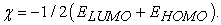(2)

For all the studied descriptors, the analysis of the bivariate data, i.e. the calculation of the partial correlation coefficient between each of the pairs of all the descriptors, is less than 0.95 (aij < 0.95), which means that these different descriptors are independent of each other 35, 36, 37, 38, 39, 40.

2.4. Estimation of the Predictive Power of a QSAR Model

The quality of a model is determined according to various statistical analysis criteria including the determination coefficient R2, the standard deviation S, the correlation coefficients of the cross-validation Q2CV and Fischer F. R2, S and F relate to the adjustment of the calculated and experimental values. They describe the predictive capacity within the limits of the model, and allow the accuracy of the calculated values on the learning game (training set) to be estimated 41, 42, 43. The cross-validation coefficient Q2CV provides information on the predictive power of the model. This forecasting power is called "internal" because it is calculated from the structures used to build this model.

The correlation coefficient R² gives an evaluation of the dispersion of the theoretical values around the experimental ones. The quality of a model is good when the points are close to the adjustment line 44. The adjustment of the points on this line can be evaluated by the determination coefficient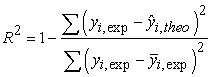(3)

Where:

y (i,exp): The experimental value of antifungal activity

y^ (i,theo): The theoretical value of the activity

y− (i,exp): The mean value (average) of the experimental values of antifungal activity

The closer the value of R² is to 1, the more the theoretical and experimental values are correlated In addition, the variance σ2 is determined by the following relationship (4):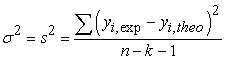(4)

Where k is the number of independent variables (descriptors), n is the number of molecules in the training test or learning set and n-k-1 is the degree of freedom.

The standard deviation S is another statistical indicator which has been used. It evaluates the reliability and precision of a model: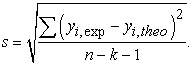(5)

The Fisher F test is also used to measure the level of statistical significance of the model, i.e. the quality of the choice of the descriptors constituting the model.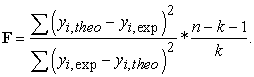(6)

The coefficient of determination of the cross-validation Q2CV, which allows to evaluate the accuracy of the prediction on the test set is calculated using the following relationship:(7)

The performance of a mathematical model, for Eriksson et al 45, 46, is characterized by a value of Q2cv > 0.5 for a satisfactory model when for the excellent model Q2cv > 0.9. According to them, for a given validation set, a model will perform well if the acceptance criterion R2- Q2cv < 0.3 is met. In addition, the predictive power of a model can be obtained from the value of the pMICtheo/pMICexp ratio for the validation set. The model is acceptable when the value of the ratio of theoretical and experimental activity tends towards unity.

### 3. Results and Discussion

3.1. QSAR Models

Table 1 lists the seven (07) molecules of the training set and the three (03) molecules of the validation set. Subsequently, the values of the partial correlation coefficients aij of the descriptors are also presented in Table 2.

The partial correlation coefficients aij between the descriptor pairs (EHO, χ), (EHO, ε0) are all less than 0.95 (aij <0.95). This demonstrates the independence of the descriptors used to develop the models.

3.2. QSAR Model Validation

It should be noted that the negative or positive sign of the descriptor’s coefficient of the model reflects the proportionality’s effect between the evolution of biological activity and this parameter of the regression equation. Thus, the negative sign indicates that when the value of the descriptor is high, the biological activity decreases while the positive sign translates the opposite effect. The best QSAR models obtained for the different antifungal activities as well as the statistical indicators are given in Table 3 and Table 4. These models were developed using the same training and validation sets as in Table 1.

The positive signs of the energy coefficients of the highest occupied molecular orbital and the negative signs of electronic energy for models 1 and 2 reflect respectively that the Aspergillus niger and Aspergillus foetidus activities will be improved for a high value of the energy of the highest occupied molecular orbital and for a low value of electronic energy. As for the negative signs of the HOMO energy coefficients and electronegativity for model 3, they reflect that the Fusarium oxysporum activity will be improved for low values of these quantum descriptors.

The significance of these models is expressed by the correlation coefficients of the cross-validation Q2CV > 0.9. These models are all acceptable with R2-Q2CV < 0.3.

The regression lines between the experimental and theoretical antifungal activities of the training sets (blue dots) and the validation sets (red dots) are illustrated in Figure 2.

• Figure 2. Regression lines of models 1, 2 and 3

External validation of models 1, 2 and 3 was performed with α-diaminophosphonates (1, 7, 9), (1, 5, 10) and (3, 5, 9) respectively. The values of the pMICtheo/pMICexp ratio of the external validation set are presented in Table 5.

All the values of the ratio pMICtheo/pMICexp are equal to 1. This indicates the good correlation between the theoretical and experimental biological activities of the studied Dapsone derivatives. These models are therefore acceptable for the prediction of the three studied antifungal activities (Aspergillus niger, Aspergillus foetidus and Fusarium oxysporum) of the series of α-diaminophosphonates derived from Dapsone.

3.3. Analysis of the Contribution of Descriptors in Models

The study of the relative contribution of descriptors in predicting the antifungal activities of compounds was carried out using XLSTAT software version 2014 34. The different contributions are presented in Figure 3.

Electronic energy is the priority descriptor in the prediction of antifungal activities Aspergillus niger and Aspergillus foetidus. In addition, global electronegative is essential to predict Fusarium oxysporum activity.

• Figure 3. Contribution of the different components in models 1, 2 and 3

### 4. Conclusion

The energy of the highest occupied molecular orbital (EHO), global electronegativity (χ), and electronic energy (ε0) allowed us to predict the antifungal activities (Aspergillus niger, Aspergillus foetidus and Fusarium oxysporum) of Dapsone derivatives. This study showed the existence of strong correlations between calculated and experimental values of antifungal potentials. The QSAR models obtained allow us to predict the activity of the best analogues called "lead". These proposed models reveal that electronic energy and global electronegativity are the priority descriptors for improving antifungal activity. This work is a compass for the design of new, more active molecules. The significance of these models was verified using a test set including three molecules. The work presented here will therefore play an important role in understanding the relationship between the physico-chemical parameters of the structure and biological activity. The study of these QSAR models could help us to select the appropriate substituent in order to design new compounds with improved biological activity.

### References

Published with license by Science and Education Publishing, Copyright © 2019 Jean Stéphane N’dri, Ahmont Landry Claude Kablan, Bafétigué Ouattara, Mamadou Guy-Richard Koné, Lamoussa Ouattara, Charles Guillaume Kodjo and Nahossé ZiaoThis work is licensed under a Creative Commons Attribution 4.0 International License. To view a copy of this license, visit http://creativecommons.org/licenses/by/4.0/